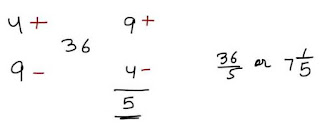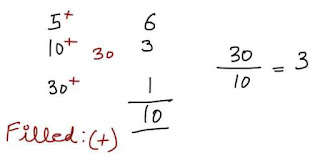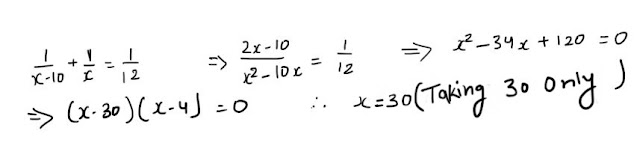# Pipes and Cisterns Shortcuts with Examples

Pipes and Cisterns is an important topic for banking exams. Today I am going to share technique to solve questions from this chapter quickly.

### Steps to Solve Pipes and Cistern with Trick

Step 1. Take the LCM of a given Number and that LCM will be the total capacity of cistern or
tank.Place the plus or minus sign for common understanding through picture. plus sign means time taken to fill the cistern and minus sign means to empty the cistern in a particular time.
Step 2. Add or Subtract According  to a particular question
Step 3. The LCM from Step 1. will be the total work . Divide the total capacity of cistern or tank with the outcome of Step 2.

## Examples

### #1.

Two pipes A and B can fill a tank in 20 and 30 minutes respectively. If both the pipes are used together, then how long will it take to fill the tank ?  (M.A.T 2003)

Step 1. Take the LCM of 20 and 30 which is 60. Sixty liter is the total capacity of tank or cistern.
Step 2. Divide 60 by (each number) 20 and 30 ,you will get 3 and 2 respectively.
Step 3. Now add  (3+2) which is 5.
Step 4. Now Divide  Total capacity of tank or cistern ( 60/5) , you will get Total time taken by them to fill the tank or cistern in a particular time which is in this case is 12 minutes.### #2.

A cistern can be filled by a tap in 4 hours while it can be emptied by another tap in 9 hours. If both the taps are opened simultaneously then after how much time will the cistern get filled ? (Hotel management, 1997)

Step 1. Take the LCM of 4 and 9 which is 36. Thirty six liter is the total capacity of tank or cistern.
Step 2. Divide 36 by (each number) 4 and 9 ,you will get 9 and 4 respectively.Here plus (+) sign means filling the tank or cistern and minus (-) implies time taken by them to empty the tank.
Step 3. Now You see one is filling the tank and other empty it so subtract it with each other.you will get (9-4) 5.
Step 4. Now Divide  Total capacity of tank or cistern ( 30/5) , you will get Total time taken by them to fill the tank or cistern in a particular time which is in this case is 36/5 minutes.### #3.

Pipe A can fill a tank in 5 hours, pipe B in 10 hours and pipe C in 30 hours. If all the pipes are open, in how many hours will the tank be filled ? (C.B.I, 1997)

Step 1. Take the LCM of 5,10 and 30 which is 30. Thirty liter is the total capacity of tank or cistern.
Step 2. Divide 30 by (each number) 5,10 and 30 ,you will get 6,3 and 1 respectively.Here plus (+) sign means filling the tank or cistern and minus (-) implies time taken by them to empty the tank.
Step 3. Now you see here each tank is filling the tank in a particular time, so add each value (6+3+1) you will get 10.
Step 4. Now Divide  Total capacity of tank or cistern ( 30/10) , you will get Total time taken by them to fill the tank or cistern in a particular time which is in this case is 3 hours.### #4.

Pipes A and b can fill a tank in 5 and 6 hours respectively. Pipe C can empty it in 12 hours. If all the three pipes are opened together, then the tank will be filled in ? (Bank P.O. 2002)## Practice Questions

### Ques 1.

Two pipes P and Q can fell a cistern in 24 min. and 36 min. respectively . Third pipe R can empty it in 36 min. If all the three pipes are opened , find the taken to fill the cistern.
(a) 1 hour
(b) 24 mins
(c) 36 mins
(d) 30 mins

### Ques 2.

A tank has a leak which would empty it in 8 hours. A tap is turned on which admit 6 liters a minute into the tank and it now emptied in 12 hours . How many literes does the tank holds?
(a) 8260 ltr
(b) 8640 ltr
(c) 8560 ltr
(d) 8800 ltr

### Ques 3.

When the waste pipe is closed , two taps can separately fill a cistern in 10 and 12 minutes respectively .when the waste pipe is opened they together fill it in 15 minutes . How long does it take waste pipe to empty the cistern , when the taps are closed?
(a) 8 min. 34 sec.
(b) 7 min.10 sec.
(c) 12 min.
(d) 10 min

### Ques 4.

Two pipes can fill a tank in 10 minutes and 30 minutes respectively and a third pipe can empty the full tank in 20 minutes . If all the three pipes are opened simultaneously, the tank will be filled in:
(a) 12 minutes
(b) 10 minutes
(c) 8 minutes
(d) 6 minutes

### Ques 5.

Two pipes can fill a cistern in 14 hours and 16 hours respectively . The pipes are opened simultaneously and it is found that due to leakage in the bottom , 32 minutes extra are taken for the cistern to be filled up. When the cistern is full , in what time will the leak empty it ?
(a) 108 hours
(b) 112 hours
(c) 116 hours
(d) 120 hours

### Ques 6.

A cistern can be filled by pipes A and B in 12 minutes and 10 minutes respectively . The full tank can be emptied by a third pipe C in 8 minutes only .If all the pipes be turned on at the same time,the cistern will be full in :
(a) 17 min
(b) 171/7 min.
(c) 17 2/7 min.
(d) 18 min.

### Ques 7.

If two pipes function simultaneously , the reservoir will be filled in 12 hours. One pipe fills the reservoir 10 hours faster than the other. How many hours does it take the second pipe to fill the reservoir?
(a) 20 hours
(b) 25 hours
(c) 30 hours
(d) 40 hours

### Ques 8.

A tank is filled in hours by three pipes A, B and C . The pipe C is twice as fast as B and B is twice as fast as A. How much time will pipe A alone take to fill the tank?
(a) 40 hours.
(b) 35 hours
(c) 30 hours
(d) 25 hours

### Ques 9.

Three pipes P , Q and R can fill a tank in 6 hours , After working it at together for 2 hours , R is closed and P and Q can fill the remaining part in 7 hours . How much time will R take alone to fill the tank?
(a) 16 hours
(b) 14 hours
(c) 12 hours
(d) 10 hours

### Ques 10.

Three taps P, Q and R can fill a tank in 12, 15 and 20 hours respectively . If P is opened all the time and Q and R are opened for one hour each alternately , the tank will be full in
(a) 5 hours
(b) 6 hours
(c) 6 2/3 hours
(d) 7 hours

### solutions:

Ans 1.Since time taken to fill the cistern by Q = time taken to empty the cistern by R so
the cistern will be filed by P in 24 min.

Ans 2. In I hour the part filled by the tap = 1/8 -1/12 = 1/24
Hence , the tap can fill the tank in 24 hours.
Therefore, capacity of the tank = 24 x 60 x 6 = 8640 litres.

Ans 3. The waste pipe can empty in 1 min .= 1/10 + 1/12 - 1/15 = 11/60 - 1/15 = 7/60
of the cistern.
Hence, the waste pipe can empty the cistern in 60/7 minutes , i.e., 8 minutes 34 sec.

Ans 4
. 1n I minute the part filled by all the three pipes = 1/10 + 1/30 - 1/20 = 1/12
Hence , all the three pipes will fill the bank in 12 minutes.

Ans 5. In I hour the part filled by both the pipes = 1/14 + 1/16 = 15/112
Hence, both the pipes will fill the cistern in 112/15 min.
but due to leakage , the tank will be filled in 112/15 + 32/60 = 120/15 = 8 hours.
Since, in 1 hr. the part emptied by the leakage = 15/112 - 1/8 = 1/112
Therefore , leakage will empty the tank in 112 hours.

Ans 6
. In 1 minute the part filled by the three pipes = 1/12 + 1/10 -1/8 = 7/120
Hence, all the three pipes will fill the cistern in 120/7 = 17 1/7 minutes.

Ans 7.Let ( x- 10) and x hrs be the time taken by two pipes to fill the reservoir , then,Hence , second pipe will fill the reservoir in 30 hours.

Ans 8.
Let x,x/2 and x/4 hours be the time taken by pipes A, B and C respectively to
fill the tank,then
1/x + 2/x + 4/x = 1/5 => 7/x = 1/5            ∴ x = 35 hours.
Hence , time taken by A is 35 hours to fill the tank.

Ans 9. In 2 hour the part filled by all the three pipes = 2 X 1/6 = 1/3
Remaining part = 1 - 1/3 = 2/3, which is filled by ( P + Q ) in 7 hours.
Hence , in 1 hour the part filled by ( P + Q ) = 2/ 3 x 7 = 2/21
Since , in 1 hour the part filled by R alone = 1/6 - 2/21= 1/14
Therefore, R alone will fill the bank in 14 hours.

Ans 10.In 1 hour the part filled by (P + Q ) = 1/12 + 1/15 = 3/20
In 1 hour the part filled by (P + R ) = 1/12 + 1/20 = 2/15
Hence, in 2 hours the part filled by ( P+ Q +R ) = 3/20 + 2/15 = 17/60
Then , in 6 hours the part filled by ( P + Q + R ) = 3 x 17/60 = 17/20
Remaining part = 1 - 17/20 = 3/20 , which is filled by ( P + Q ) in 1 hour.
Hence, total time taken = 6 + 1 = 7 hours.

Updated on 18 February 2016#### What's trending in BankExamsToday

Smart Prep Kit for Banking Exams by Ramandeep Singh - Download here1.Thanks exactly what I required thanks

2.Time saving method sirji thanks a lot

3.Thanku sir

Sir boat and stream nahe bnta hai iske liye kch help kijye sir

4.SIR BOAT AND STEAM KA V DIAJIYY PLS

5.thnx sir very usefullll

6.7.@ramandeep Sir
In problem 2 step 1
How can we calculate capacity of cistern in hours??

8.Sir plz provide practice set for mixture and alligation as i m weak in that chapter

9.Thank you so much.......sir.........you are awesome....

10.Agr 2taps every hours ek dusre k bad open ho to question kaise hoga

11.work with the total capacity of 2 taps required 2 hours.

12.thank u sir:) really easiest method:)

13.thankyou, thankyou, thankyou very much thanks sir.........
it's really good

14.Thank u sir.. u made this topic so easy..

15.Itne easy ques exm me nhi aate hai...plz provide tricks for tough and lenthy ques...thanx

16.